# GRE Quantitative: Algebra – Graphs of Functions

On the day of your GRE exam , you should be certain that you will have to attempt a few questions based on functions. Hence, ignoring the topics of functions can adversely affect your performance in GRE. But being comfortable with functions and its graphs will not only help you solve the problems based on functions but on coordinate geometry as well.

Before we move on to any complex definitions or graphs, let us first understand what functions actually mean. Functions can be imagined as a machine that has an input and an associated output. There is always a rule through which output and input can be related. The classical abbreviation used for representing functions is f(x)=…

There are many standard functions whose graphs are essential for the GRE Quantitative Reasoning section and that needs proper practice. We will understand these functions and their graphs one at a time.

1. Linear Function: The rule used for presenting a linear function is represented as y = mx + b. It is the equation of a line as lines are always linear in nature. Here, in this equation, y represents how far up the line went; x represents how far along the line is, m is the gradient or slope of the line, that is, the steepness of the line is represented through m and b is the point where the line intersects the y- axis.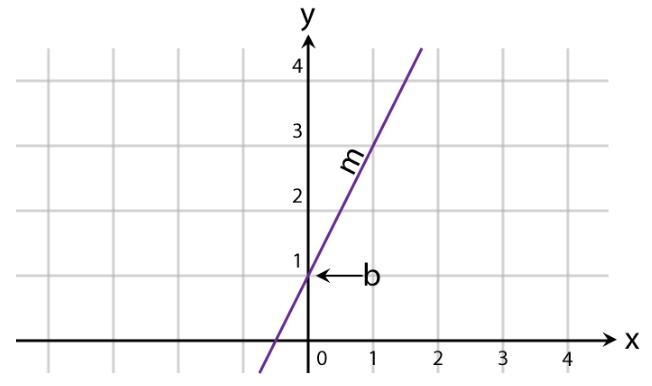1. Square Function: It is a quadratic function. The graph of y = x2 which is a graph that is symmetrical about the y-axis. Similarly, the graph of y= -x2 will be symmetrical about –y- axis. And the graphs of x= y2 and x = -y2, will be symmetrical about x- axis. The domain of quadratic function is Real number, R. Whereas, its range is positive real numbers.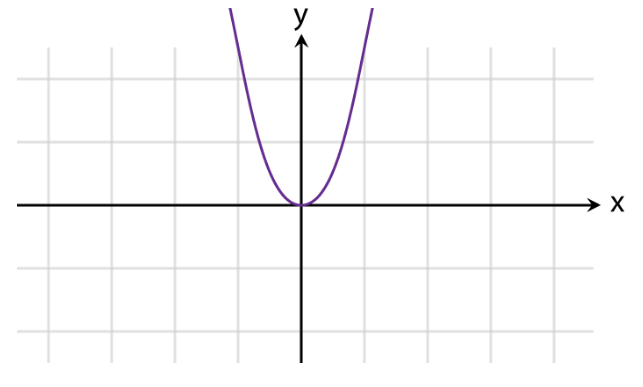1. Cubic Function: y= x3 is a cubic function in nature. The range and domain of cubic function is real numbers, R.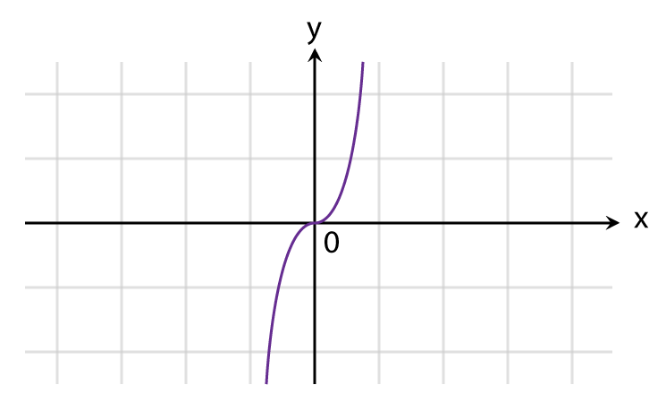1. Square root function: The equation of square root function is f(x)= x. And the domain and range of square root function is non-negative real numbers, that is [0, infinity).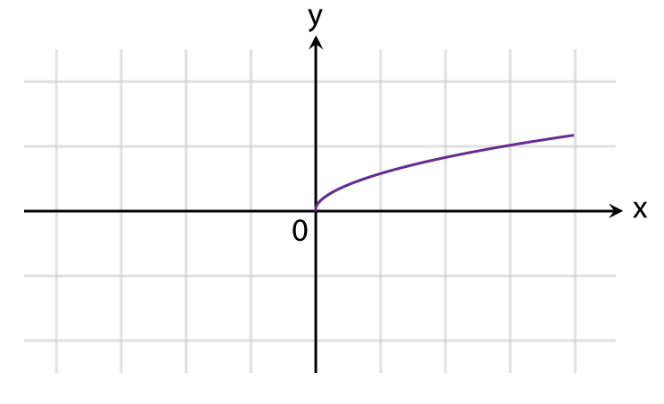1. Absolute Value Function: The equation of absolute value function is f(x) = |x|. Its range is non-negative real numbers while its domain is the whole set of real numbers.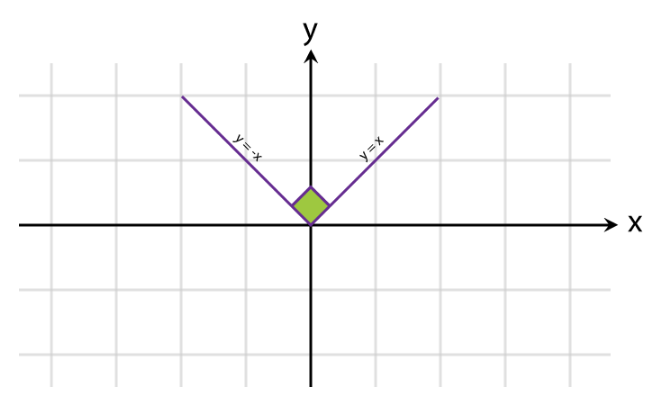1. Reciprocal Function: The equation for presenting the reciprocal function is y= 1x . The graph of reciprocal functions forms a hyperbola and it is an odd function.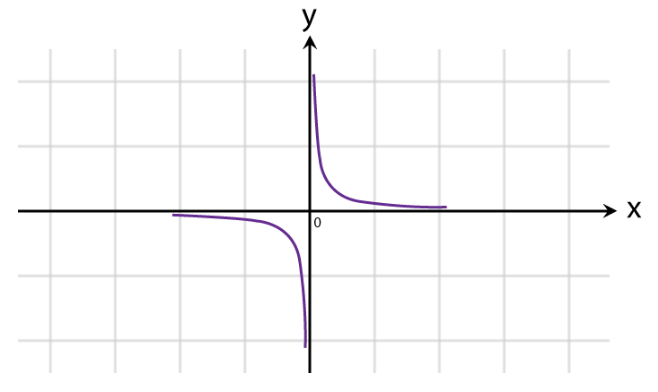1. Logarithmic Function: The equation for logarithmic function is y = loga(x). Its domain contains positive real numbers and range is made from real numbers.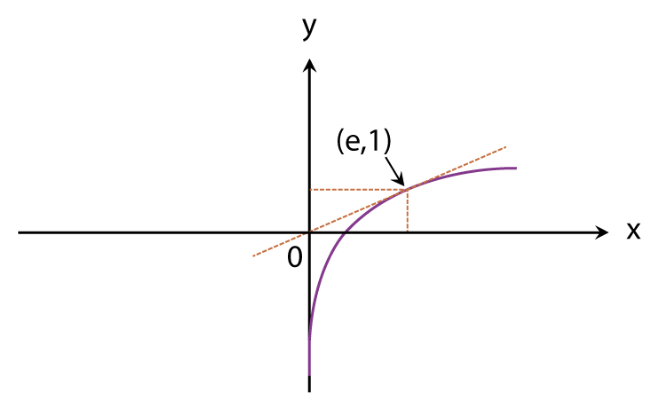1. Exponential Function: Equation- y = ax, where a is always greater than 0.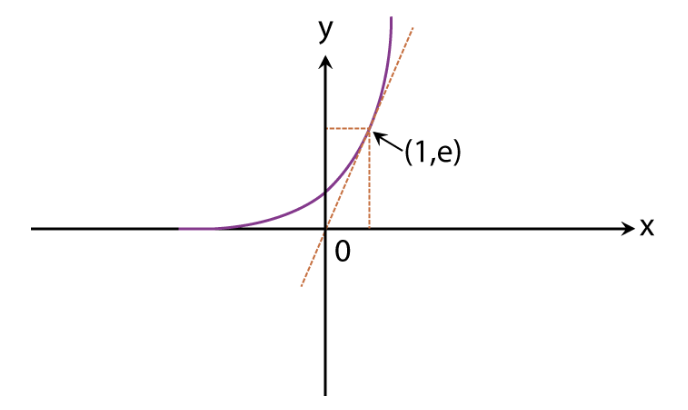1. Floor and Ceiling Function: The floor and ceiling value gives you the nearest integer value, either up or down.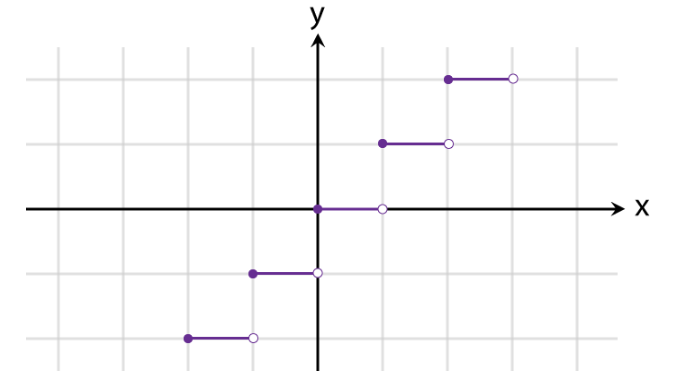1. Sine Function: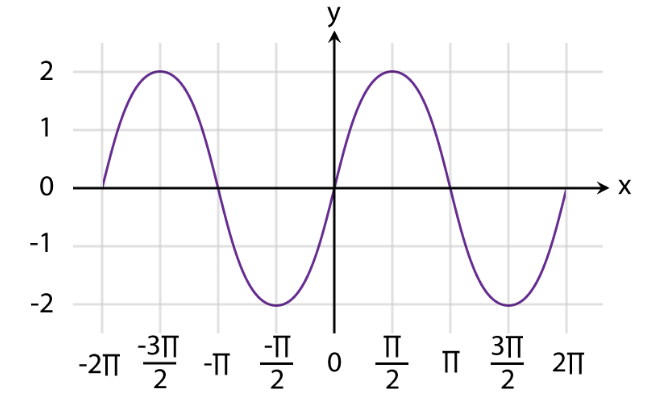1. Cosine Function: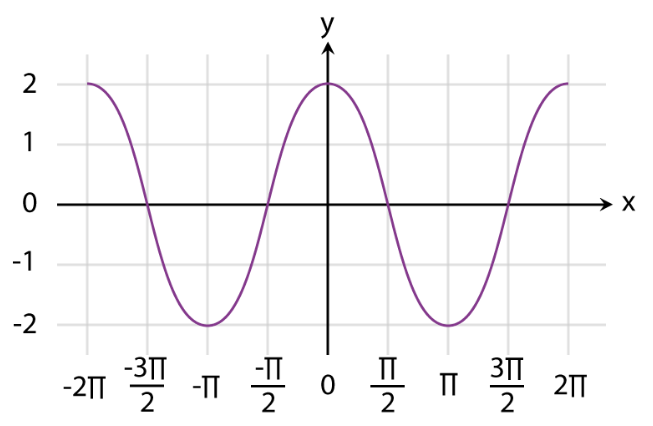1. Tangent Function: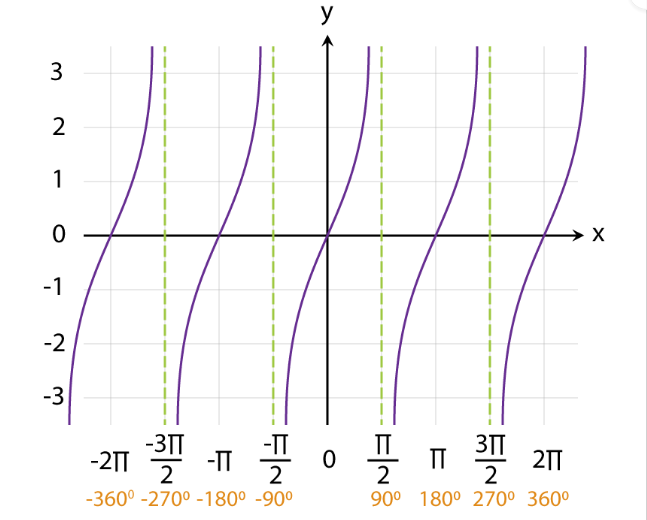BYJU’S will be glad to help you in your GRE preparation journey. You can ask for any assistance related to GRE from us by just giving a missed call at +918884544444, or you can drop an SMS. You can write to us at gre@byjus.com.